877-601-6284
Get Started by Finding a Local Center

# All About 2D Polygon Shapes

Feb 19, 2020 | Location Hinsdale

### Today's information on 2D polygon shapes comes to us from KidsMathGamesOnline (with some help from Google images!)

• A polygon is a plane (2 dimensional) figure that features at least 3 straight sides and angles.

• A regular polygon has sides which are all the same length as well as angles which are all the same. Examples of regular polygons include the equilateral triangle and square.

• triangle is a 3 sided polygon with interior angles that add to 180 degrees.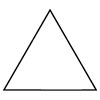• square is a 4 sided polygon with interior angles that add to 360 degrees. A square is also a type of quadrilateral.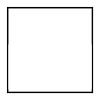• A pentagon is a 5 sided polygon with interior angles that add to 540 degrees.

• Regular pentagons have sides of equal length and interior angles of 108 degrees.

• The US Department of Defense headquarters is named 'the Pentagon' .

• The edible plant okra is shaped like a pentagon.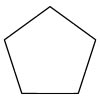• A hexagon is a 6 sided polygon with interior angles that add to 720 degrees.

• Regular hexagons have sides of equal length and interior angles of 120 degrees.

• Beehive cells are hexagonal.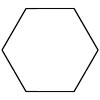• A heptagon is a 7 sided polygon with interior angles that add to 900 degrees.

• Regular heptagons have sides of equal length and interior angles of 128.57 degrees.

• The British 50 and 20 pence coins are curved heptagons.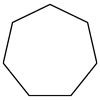• An octagon is an 8 sided polygon with interior angles that add to 1080 degrees.

• Regular octagons have sides of equal length and interior angles of 135 degrees.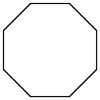• A nonagon is a 9 sided polygon with interior angles that add to 1260 degrees.

• Regular nonagons have sides of equal length and interior angles of 140 degrees.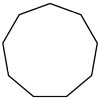• A decagon is a 10 sided polygon with interior angles that add to 1440 degrees.

• Regular decagons have sides of equal length and interior angles of 144 degrees.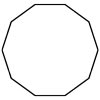• An pentadecagon is a 15 sided polygon with interior angles that add to 2340 degrees.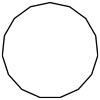• An icosagon is a 20 sided polygon with interior angles that add to 3240 degrees.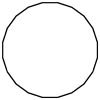##### Locations near
Could not find Center, try again